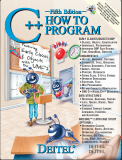Back to www.deitel.comISBN: 0-13-185757-6 © 2005 pages: 1500 Buy the Book![Note: This tutorial is an excerpt (Sections 23.1) of Chapter 23, Standard Template Library (STL), from our textbook C++ How to Program, 5/e. These tutorials may refer to other chapters or sections of the book that are not included here. Permission Information: Deitel, Harvey M. and Paul J., C++ HOW TO PROGRAM, ©2005, pp.1112-1123. Electronically reproduced by permission of Pearson Education, Inc., Upper Saddle River, New Jersey.]

23.1.3 Introduction to Algorithms (Continued)

Figure 23.11 shows many of the mutating-sequence algorithms—i.e., the algorithms that result in modifications of the containers to which the algorithms are applied.

 Mutating-sequence algorithms `copy` `remove` `reverse_copy` `copy_backward` `remove_copy` `rotate` `fill` `remove_copy_if` `rotate_copy` `fill_n` `remove_if` `stable_partition` `generate` `replace` `swap` `generate_n` `replace_copy` `swap_ranges` `iter_swap` `replace_copy_if` `transform` `partition` `replace_if` `unique` `random_shuffle` `reverse` `unique_copy`

Fig. 23.11 Mutating-sequence algorithms.

Figure 23.12 shows many of the nonmutating sequence algorithms?i.e., the algorithms that do not result in modifications of the containers to which they are applied.

 Nonmutating sequence algorithms `adjacent_find` `find` `find_if` `count` `find_each` `mismatch` `count_if` `find_end` `search` `equal` `find_first_of` `search_n`

Fig. 23.12 Nonmutating sequence algorithms.

Page 1 | 2 | 3 | 4 | 5 | 6 | 7 | 8 | 9 | 10 | 11 | 12

Tutorial Index